Normal view MARC view ISBD view

The nuts and bolts of proofs: an introduction to mathematical proofs

Material type:BookPublisher: Amsterdam Elsevier Academic Press 2013Edition: 4th ed.Description: ix, 283 p.ISBN: 9780123822178.DDC classification: 511.36 Summary: The Nuts and Bolts of Proofs: An Introduction to Mathematical Proofs provides basic logic of mathematical proofs and shows how mathematical proofs work. It offers techniques for both reading and writing proofs. The second chapter of the book discusses the techniques in proving if/then statements by contra-positive and proofing by contradiction. It also includes the negation statement, and/or. It examines various theorems, such as the if and only-if, or equivalence theorems, the existence theorems, and the uniqueness theorems. In addition, use of counter examples, mathematical induction, composite statements including multiple hypothesis and multiple conclusions, and equality of numbers are covered in this chapter. The book also provides mathematical topics for practicing proof techniques. Included here are the Cartesian products, indexed families, functions, and relations. The last chapter of the book provides review exercises on various topics. Undergraduate students in engineering and physical science will find this book invaluable. https://www.elsevier.com/books/the-nuts-and-bolts-of-proofs/cupillari/978-0-12-382217-8
Tags from this library: No tags from this library for this title.
average rating: 0.0 (0 votes)
Item type Current location Item location Collection Call number Status Date due Barcode
Books Vikram Sarabhai Library
Slot 1355 (0 Floor, East Wing) Non-fiction 511.36 C8N8-2013 (Browse shelf) Available 180869
Browsing Vikram Sarabhai Library Shelves , Collection code: Non-fiction Close shelf browser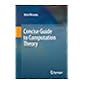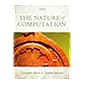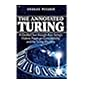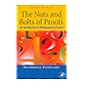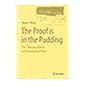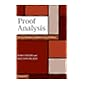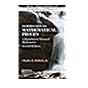511.352 M2C6 Concise guide to computation theory 511.352 M6N2 The nature of computation 511.352 P3A6 The annotated Turing: a guided tour through Alan Turing's historic paper on computability and the Turing machine 511.36 C8N8-2013 The nuts and bolts of proofs: an introduction to mathematical proofs 511.36 K7P7 The proof is in the pudding: the changing nature of mathematical proof 511.36 N3P7 Proof analysis: a contribution to Hilbert's last problem 511.36 R6I6 Introduction to mathematical proofs: a transition to advanced Mathematics

The Nuts and Bolts of Proofs: An Introduction to Mathematical Proofs provides basic logic of mathematical proofs and shows how mathematical proofs work. It offers techniques for both reading and writing proofs. The second chapter of the book discusses the techniques in proving if/then statements by contra-positive and proofing by contradiction. It also includes the negation statement, and/or. It examines various theorems, such as the if and only-if, or equivalence theorems, the existence theorems, and the uniqueness theorems. In addition, use of counter examples, mathematical induction, composite statements including multiple hypothesis and multiple conclusions, and equality of numbers are covered in this chapter. The book also provides mathematical topics for practicing proof techniques. Included here are the Cartesian products, indexed families, functions, and relations. The last chapter of the book provides review exercises on various topics. Undergraduate students in engineering and physical science will find this book invaluable.

https://www.elsevier.com/books/the-nuts-and-bolts-of-proofs/cupillari/978-0-12-382217-8

There are no comments for this item.